# Go Math Grade 3 Answer Key Chapter 8 Understand Fractions Extra Practice

Free step by step solutions to Go Math Grade 3 Answer Key Chapter 8 Understand Fractions Extra Practice. Build fluency with the math standards by solving the 3rd Grade Go Math Answer Key Chapter 8. Students who want to score good marks in the exams can start your preparation now. You can solve the Extra Practice Questions here and check the answers provided in the Go Math Grade 3 Answer Key Chapter 8 Understand Fractions.

## Go Math Grade 3 Answer Key Chapter 8 Understand Fractions Extra Practice

Practice more and more to improve knowledge in this subject. If you understand the concept you can make a question on your own and solve them. Resources are available to support your child’s learning in our Go Math Answer Key.

### Common Core – Page No. 171000

Lesson 8.1

Write the number of equal parts. Then write the name for the parts.

Question 1.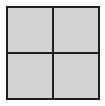Equal parts: _________
Name: _________

i. 4
ii. Fourths

Explanation:

The figure shows that the square is divided into 4 equal parts. So, the name for the parts is fourths.

Question 2.Equal parts: _________
Name: _________

i. 2
ii. Halves

Explanation:

The circle is divided into two equal parts. Thus the name for the parts is halves.

Question 3.Equal parts: _________
Name: _________

i. 6
ii. Sixths

Explanation:

The hexagon is divided into six equal parts. Thus the name for the parts is sixths.

Lesson 8.2

Draw lines to show how much each person gets. Write the answer.

Question 4.
4 friends share 3 oranges equally.Type below:
___________

Answer: 3 fourths of an orange

Explanation:

There are 3 oranges and
The total number of friends = 4
We need to divide the 3 oranges for 4 friends equally
= 3/4
So, each friend gets 3 fourth of the orange.

Question 5.
6 sisters share 4 sandwiches equally.Type below:
___________

Answer: 4 sixths of a sandwich

Explanation:

Total number of sandwiches = 4
Number of friends = 6
To share the sandwiches equally we need to divide the number of sandwiches by a number of friends.
4/6
So, each friend gets 4 sixths of the sandwich.

Lessons 8.3–8.4

Write the number of equal parts in the whole. Write a fraction in words and in numbers to name the shaded part.

Question 6.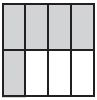_________ equal parts
_________ eighths

Question 6.
$$\frac{□} {□}$$

i. 8
ii. Five
iii. $$\frac{5} {8}$$

Explanation:

The square is divided into 8 eight equal parts.
Among them, five parts are shaded.
So, the fraction name for the shaded part is $$\frac{5} {8}$$.

Question 7.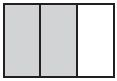_________ equal parts
_________ thirds

Question 7.
$$\frac{□} {□}$$

i. 3
ii. Two
iii. $$\frac{2} {3}$$

Explanation:

The aboved figure shows that the rectangle is divided into 3 equal parts. And 2 parts are shaded among them.
The fraction name for the shaded part is 2 thirds i.e, $$\frac{2} {3}$$

### Common Core – Page No. 172000

Lesson 8.5

Write the fraction that names the point.Question 1.
point A: $$\frac{□} {□}$$

Answer: $$\frac{1} {6}$$

Explanation:

The number lies between 0 and 1. Each point is 1 sixth of the number line. So, it starts with 0/6 and ends on 6/6.
Now we have to locate the point A.
The point A lies between 0/6 and 2/6. The number between 0 and 2 is 1.
Thus the point A is $$\frac{1} {6}$$

Question 2.
point B: $$\frac{□} {□}$$

Answer: $$\frac{3} {6}$$

Explanation:

The point B lies between $$\frac{2} {6}$$ and $$\frac{4} {6}$$. The number between 2 and 4 is 3. Thus the location of point B is $$\frac{3} {6}$$.

Question 3.
point C: $$\frac{□} {□}$$

Answer: $$\frac{5} {6}$$

Explanation:

The point C is located between $$\frac{4} {6}$$ and $$\frac{6} {6}$$.
The number between 4 and 6 is 5. Thus the location of Point C is $$\frac{5} {6}$$

Lesson 8.6

Each shape is 1 whole. Write a fraction greater than 1 for the parts that are shaded.

Question 4.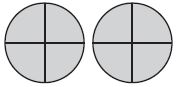2 = $$\frac{□} {□}$$

Answer: $$\frac{8} {4}$$

Explanation:

There are 2 circles and each circle is equally divided into 4 groups.
Number in each group = 4
Number of equal parts = 8
The shaded part of the circle = $$\frac{8} {4}$$

Question 5.3 = $$\frac{□} {□}$$

Answer: $$\frac{18} {6}$$

Explanation:

Number of circles = 3
Number of equal parts = 18
Number of shaded part in each circle = 6
So, the fraction of the shaded part is $$\frac{18} {6}$$

Lesson 8.7

Write a fraction to name the shaded part of each group.

Question 6.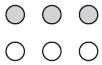$$\frac{□} {□}$$

Answer: $$\frac{3} {6}$$ or $$\frac{1} {2}$$

Explanation:

There are 6 circles, in which 3 circles are shaded and 3 circles are not shaded.
So, the fraction name for the shaded part is $$\frac{3} {6}$$ or $$\frac{1} {2}$$

Question 7.$$\frac{□} {□}$$

Answer: $$\frac{1} {4}$$ or $$\frac{2} {8}$$

Explanation:

The figure shows that there are 8 circles and in that 2 circles are shaded.
So, the fraction name for the shaded part is $$\frac{2} {8}$$ or $$\frac{1} {4}$$

Lessons 8.8–8.9

Draw a quick picture to solve.

Question 8.
Charlotte has 12 T-shirts. One-fourth of her T-shirts is green. How many of Charlotte’s T-shirts are green?
_________ green T-shirts

Explanation:

Given,
Charlotte has 12 T-shirts.
One-fourth of her T-shirts is green.
To know how many of Charlotte’s T-shirts are green we need to multiply 12 with 1/4
12 × $$\frac{1} {4}$$
12/4 = 3
Therefore there are 3 green T-shirts.

Question 9.
Josh walks 18 dogs each week. Today, he is walking $$\frac{1}{3}$$ of the dogs. How many dogs is he walking today?
_________ dogs

Explanation:

Given that Josh walks 18 dogs each week.
Today, he is walking $$\frac{1}{3}$$ of the dogs.
To find how many dogs he walked today
We need to multiply 18 and $$\frac{1}{3}$$
18 × $$\frac{1}{3}$$
= 18/3 = 6
Thus he walked 6 dogs today.

The Go Math Grade 3 Answer Key Chapter 8 Understand Fractions Extra Practice helps to test skills in this chapter. The understanding fractions chapter includes various topics like Equal parts of a whole, unit fractions, relate fractions and whole numbers. We have provided the images for the questions for understanding. Quick and easy learning of maths is possible on our Go Math Answer Key.

Scroll to Top